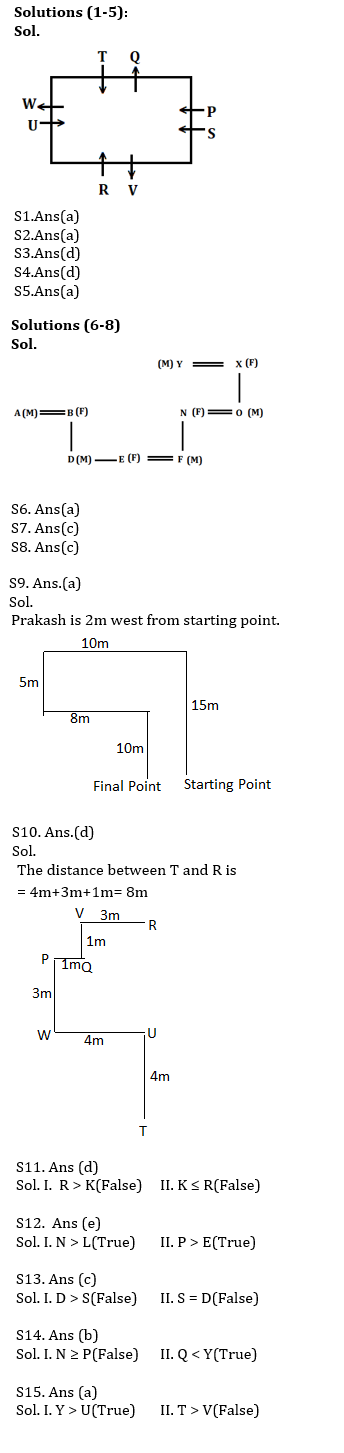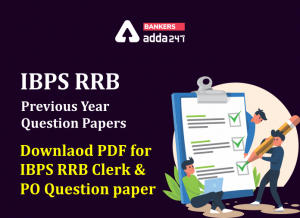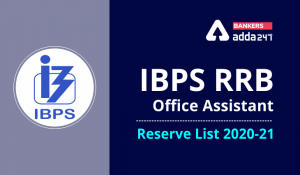Latest Banking jobs   »   Reasoning Ability Quiz For IBPS RRB...

# Reasoning Ability Quiz For IBPS RRB PO, Clerk Prelims 2021- 23rd May

Directions (1-5): Study the information carefully and answer the questions given below.
Eight persons P, Q, R, S, T, U, V and W are sitting around a rectangular table in such a way that two persons sit on each side of the table. Some of them are facing towards the center while some of them are facing away from the center. Not more than two persons sitting together face same direction.
T sits second to the right of P. W sits second to the left of R and W is not an immediate neighbor of P. R faces T. S sits fourth to the right of W. V sits second to the right of U. W and V do not sit on the same side of the table. R sits to the immediate right of V. W faces opposite direction of S, who face inside.

Q1. How many persons facing inside?
(a) Five
(b) Two
(c) Four
(d) Three
(e) None of these

Q2. Which of the following pair of persons are immediate neighbor of R?
(a) U-V
(b) P-U
(c) Q-U
(d) V-S
(e) None of these

Q3. How many persons are sitting between W and P, when counted right of P?
(a) One
(b) Four
(c) Three
(d) Two
(e) None

Q4. Which of the following statement is true about T?
(a) T faces outside
(b) Two persons sit between T and S, when counted right of T
(c) R sits immediate left of T
(d) T is immediate right of W
(e) None is true

Q5. Four of the following five are alike in certain way based from a group, find the one that does not belong to that group?
(a) W
(b) U
(c) P
(d) S
(e) T

Directions (6-8): Study the following information carefully and answer the given questions:
In a family there are nine members. Y is the grandfather of F, who is brother in law of D, who is the only son of A. E is the daughter in law of N, who is the daughter in law of X. B is the mother in law of F, who is son of O.

Q6. How is X related with F?
(a) Grandmother
(b) Granddaughter
(c) Mother
(d) Father
(e) None of these

Q7. How is B related with D?
(a) Son in law
(b) Daughter in law
(c) Mother
(d) Granddaughter
(e) Cannot be determined

Q8. How many male members are there in the family?
(a) Four
(b) Three
(c) Five
(d) Two
(e) None of these

Q9. Prakash starts walking towards his office. He walks 15 m towards north and then 10 m towards west. He then turned to the south and covered 5 m. Further, he turned to the east and moved 8 m. Finally, he turned right and walks 10 m. How far and in which direction is he from his starting point?
(a) 2 m West
(b) 5 m East
(c) 6 m South
(d) 3 m South
(e) 2 m east

Q10. Gaurangi walks in the north direction from point T to reach point U, which is 4m away. From point U she takes a left turn and reach point W which is 4m away and then takes a right turn and walks 3m to reach point P, again he takes a right turn and walks 1m to reach point Q. From point Q he takes a left turn to reach point V, which is 1m away and finally takes a right turn and walks for point R, which is 3m away. What is the distance between T and R?
(a) 4m
(b) 5m
(c) 7m
(d) 8m
(e) None of these

Directions (11-15): In these questions, relationship between different elements is shown in the statements. These statements are followed by two conclusions:

Q11.
Statements:
K ≤ M < B ≥ W < R ≥ S = T
Conclusion:
I. R > K
II. K ≤ R
(a) If only conclusion I follows.
(b) If only conclusion II follows.
(c) If either conclusion I or II follows.
(d) If neither conclusion I nor II follows.
(e) If both conclusion I and II follow.

Q12.
Statements:
R > L ≤ U < N > P = T > E
Conclusion:
I. N > L
II. P > E
(a) If only conclusion I follows.
(b) If only conclusion II follows.
(c) If either conclusion I or II follows.
(d) If neither conclusion I nor II follows.
(e) If both conclusion I and II follow.

Q13.
Statement:
W ≥ D = O ≥ T ≥ E ≥ S < R
Conclusions:
I. D > S
II. S = D
(a) If only conclusion I follows.
(b) If only conclusion II follows.
(c) If either conclusion I or II follows.
(d) If neither conclusion I nor II follows.
(e) If both conclusion I and II follow.

Q14.
Statement:
M ≤ N > Y = T > O ≥ P = Q
Conclusions:
I. N ≥ P
II. Q < Y
(a) If only conclusion I follows.
(b) If only conclusion II follows.
(c) If either conclusion I or II follows.
(d) If neither conclusion I nor II follows.
(e) If both conclusion I and II follow.

Q15.
Statement:
U ≤ V < W =X < Y ≥ T ≤ I
Conclusions:
I. Y > U
II. T > V
(a) If only conclusion I follows.
(b) If only conclusion II follows.
(c) If either conclusion I or II follows.
(d) If neither conclusion I nor II follows.
(e) If both conclusion I and II follow.

Practice More Questions of Reasoning for Competitive Exams:

###### Study Plan for IBPS RRB PO/Clerk Prelims 2021

Solutions#### Congratulations!Incorrect details? Fill the form again here

•IBPS RRB Previous Year Question Paper PD...
•IBPS RRB Clerk 2nd Reserve List 2022 Out...
•Reasoning Ability Quiz For IBPS RRB PO P...
•Quantitative Aptitude Quiz For IBPS RRB ...
•Reasoning Ability Quiz For IBPS RRB PO P...
•Quantitative Aptitude Quiz For IBPS RRB ...